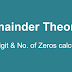## REMAINDER THEOREM, Unit Digit, and Number of Zeros

Find the number in Unit place of following:

1) (623)^49
2) (98)^43
3) (122)^20

Find the number of zeros in the following expressions:

1) 47!
2) 144! * 5 * 15 * 22 * 11 * 44 * 135
3) 1142! * 348 ! * 17!
4) 1^1 * 2^2 * 3^3 * 4^4 *......* 49^49
5) 1^1! * 2^2! * 3^3! * 4^4! *......* 10^10!

Find the remainder when

1) 17 * 23 is divided by 12
2) 37 * 43 * 51 is divided by 7
3) 43^197 is divided by 7
4) 21^875 is divided by 17

In this post, we are going to learn how to deal with these type of questions in depth.

### How To Find UNIT DIGIT of a Mathematical expression:

• If we raise any number to the power 4n, the unit digit of the new number comes  as 1, 5 or 6.
• Any number raised to the power 4n repeats its unit digit,
• e.g. Unit digit of (3^4)^12 = (3^4)^22 = (3^4)^99 = (3^4)^234556
• Unit digit powered to 4n times:
1,3,7, 9     - 1
5                - 5
2,4,6,8      - 6

Question: Find the Unit digit of (33)^123

Solution: for unit digit we can write 3^123 => (3^4)^30 * 3^3 => 1*3^3 => 7

Question: Find the Unit digit of (623)^49

Solution: for unit digit we can write 3^49 => (3^4)^12 * 3^1 => 3^1 =3

### How To Find NUMBER OF ZEROS of a Mathematical expression:

Zeros are formed by the  combination of 2 * 5, the total number of pairs of 2 and 5 makes zeros in an expression. (Apart from multiples of 10)

To find the number of 2's and 5's we need to factorize the given mathematical expression.

It is to be noted here that in factorial forms the number of 5's will always be lesser than 2's. Hence, we just need to count the number of 5's.

Question: Find the number of zeros in 6!

solution: 6! = 6 * 5 * 4 * 3 * 2 * 1  = (3*2)* 5 * 2*2 * 3 * 2 * 1

This expression contains only one pair of 5 and 2, so only one zero.

Question: Find the number of zeros in 47!.

Solution: 47/5 = 9 (quotient)

9/5 =  1

Hence total 10 zeros.

Question: Find the number of zeros in 1^1 * 2^2 * 3^3 * 4^4 *......* 49^49.

Solution: The 5's will be lesser than the 2's therefore we need to count                         a number of 5's only.

Thus: 5^5 * 10^10 * 15 ^15 * 20^20* 25^25 * 30^30 * 35^35 *                  40 ^ 40 * 45 ^ 45
=> 5+10+15+20+50+ 30+35+40+45 => 250 zeros

(25 means 5 * 5)#

### REMAINDER THEOREM:

Question: Find the remainder when 17 * 23 is divided by 12

Solution :  (12+5)*(12+11) / 12

In this type of questions  remainder only depends on the last term

=> 5*11 / 12  => 7

Question: Find the remainder when 37 * 43 * 51 is divided by 7

Solution : 2*1*2 / 7 => 4

Negative remainder

Consider the following case

14 * 15 /8 => 6*7 /8 => 2

However here we can use negative remainder

When 14 is normally divided by 8 remainders is seen as +6, however, there might be a time where negative remainder can be more useful.

Remainders, by concepts, are always positive, hence when we divide -27 by 5 we say that remainder is 3 and not (-2). however we can use the negative remainder to ease our calculations.

Thus above example may be solved as  -2 * -1  => 2, which is same but less calculative.

If in this case answer comes out to be negative you need to subtract it with original number. e.g. (62 * 63 * 64)/66   => (-4*-3*-2 ) =>-24

Hence, required remainder will be 66-24 = 42

### How to Find Remainder while Dealing with Large Powers:

1) If the expression can be expressed in the form of { (ax+1)^n } / a, the remainder will become 1 directly, the value of the power does not matter here.

e.g. 46^32624 / 9 => 1^32624 => 1

2) If the expression can be expressed in the form of { (ax-1)^n } / a , then
if n is even then, the remainder will be +1,
or n is odd then, the remainder will be -1.

e.g. 35^243 /9 => (-1)^243 => -1 => hence remainder = 8

Question: Find the remainder when 43^197 is divided by 7

Solution : 43^197 /7 => 1^197 => 1

Question: Find the remainder when 4^875 is divided by 17

Solution : 4^875 /17  => (4^4)^218 * 4^3 / 17 =>  16^218 * 64/17 => 13

Remainder Theorem, Unit Digit, and Number of Zeros in expressionsReviewed by Admin on 12:35:00 PM Rating: 5